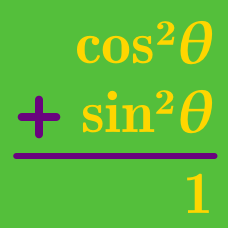Geometry

# Pythagorean Identities: Level 2 Challenges

Simplify: $\sqrt{1 - \sin^2 \theta}.$

$\large \frac { \sin ^{ 2 }{ \theta } }{ 5 } =\frac { \cos ^{ 2 }{ \theta } }{ 6 }$

If $$\theta$$ is a positive acute angle that satisfies the equation above, find $$\sin { \theta }$$.

Letting $P=\sin A \sin B \\ Q= \sin C \cos A \\ R = \sin A \cos B \\ S=\cos A \cos C$

Find the value of $$5(P^2+Q^2+R^2+S^2)$$ .

$\large\frac { 1 }{ \cos ^{ 2 }{ \theta } } +\frac { 1 }{ 1+\sin ^{ 2 }{ \theta } } +\frac { 2 }{ 1+\sin ^{ 4 }{ \theta } } +\frac { 4 }{ 1+\sin ^{ 8 }{ \theta } }$

If $$\large \sin ^{ 16 }{ \theta } = \frac { 1 }{ 5 }$$, what is the value of the expression above?

A triangle has sides of magnitude $$1$$, $$\sin x$$, and $$\cos x$$.

where $$0 < x < \frac { \pi }{ 2 } .$$

Find the largest angle of the triangle in degrees.

Assume that the triangle is non-degenerate.

×